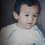# Help,its so hard for me

Determine the biggest integers 85-digit number which satisfies the sum of all digits equal to the multiplication of all numbersNote by Valian Fil Ahli
7 years, 7 months ago

This discussion board is a place to discuss our Daily Challenges and the math and science related to those challenges. Explanations are more than just a solution — they should explain the steps and thinking strategies that you used to obtain the solution. Comments should further the discussion of math and science.

When posting on Brilliant:

• Use the emojis to react to an explanation, whether you're congratulating a job well done , or just really confused .
• Ask specific questions about the challenge or the steps in somebody's explanation. Well-posed questions can add a lot to the discussion, but posting "I don't understand!" doesn't help anyone.
• Try to contribute something new to the discussion, whether it is an extension, generalization or other idea related to the challenge.
• Stay on topic — we're all here to learn more about math and science, not to hear about your favorite get-rich-quick scheme or current world events.

MarkdownAppears as
*italics* or _italics_ italics
**bold** or __bold__ bold
- bulleted- list
• bulleted
• list
1. numbered2. list
1. numbered
2. list
Note: you must add a full line of space before and after lists for them to show up correctly
paragraph 1paragraph 2

paragraph 1

paragraph 2

[example link](https://brilliant.org)example link
> This is a quote
This is a quote
    # I indented these lines
# 4 spaces, and now they show
# up as a code block.

print "hello world"
# I indented these lines
# 4 spaces, and now they show
# up as a code block.

print "hello world"
MathAppears as
Remember to wrap math in $$ ... $$ or $ ... $ to ensure proper formatting.
2 \times 3 $2 \times 3$
2^{34} $2^{34}$
a_{i-1} $a_{i-1}$
\frac{2}{3} $\frac{2}{3}$
\sqrt{2} $\sqrt{2}$
\sum_{i=1}^3 $\sum_{i=1}^3$
\sin \theta $\sin \theta$
\boxed{123} $\boxed{123}$

## Comments

Sort by:

Top Newest

I'm not sure about the answer, but I think this will help you.

Let the digits be $a_{85}\geq a_{84}\geq \cdots \geq a_1$ such that $a_1+a_2+\cdots+a_{85}=a_1a_2\cdots a_{85}$, then $\frac{a_1}{a_1a_2\cdots a_{85}}+\frac{a_2}{a_1a_2\cdots a_{85}}+\cdots+\frac{a_{85}}{a_1a_2\cdots a_{85}}=1.$ we have 85 fractions and the greatest of them is the last one, therefore $\frac{a_{85}}{a_1a_2\cdots a_{85}}\geq \frac{1}{85}$ and then $a_1a_2\cdots a_{84}\leq85$.

At most six digits among $a_1,a_2,\ldots, a_{84}$ are greater than 1, therefore, at least 78 digits are equal to 1.

- 7 years, 7 months ago

Log in to reply

Can you please explain the last sentence in your argument? It is not very obvious to me. I think we need to find the integer less than or equal to $85$ with highest number of divisors with one digit and I think according to your argument such integer has at most $6$ one digit divisors. Right?

- 7 years, 7 months ago

Log in to reply

Each of $a_1, a_2, \ldots, a_{84}$ is a positive integer (zeros are not allowed). Suppose seven digits among $a_1, a_2, \ldots, a_{84}$ are greater than 1, i.e. are $\geq2$, then the product $a_1a_2\cdots a_{84}$ will be $\geq 2^7=128$ which is a contradiction. Therefore, at most six digits among $a_1, a_2, \ldots, a_{84}$ can be greater than 1, thus, at least 78 of them are equal to 1.

- 7 years, 7 months ago

Log in to reply

Ohh.. I see.. brilliant! Thanks :)

- 7 years, 7 months ago

Log in to reply

8322111111111111111111111111111111111111111111111111111111111111111111111111111111111

highest 1 I got............ only 1 I got

8322 with 81 1s at the back

- 7 years, 7 months ago

Log in to reply

Could you show us your proof of your answer Rohan?? ^^

- 7 years, 7 months ago

Log in to reply

8x3x2x2=96.Since the other numbers are 1s, when they are multiplied by any number, it equals to the number you multiplied it by. 8+3+2+2=15, there are 81 1s that add up to 81. 81+15 =96. I hope this helped!

- 7 years, 7 months ago

Log in to reply

I think he means to say ''prove that this is the largest number and there is no other larger number''. I think a simple case by case analysis( of a lot of cases) should suffice.

- 7 years, 7 months ago

Log in to reply

I said it was the highest I got, not the answer

- 7 years, 7 months ago

Log in to reply

It is pretty easy to see why there must be at least 79 or more '1's in this number. A really interesting problem.

- 7 years, 7 months ago

Log in to reply

Could you show us your proof of this fact?

- 7 years, 7 months ago

Log in to reply

Let there be less than or equal to 78 '1's then the product of the remaining digits is at least 222..*2 (product of 7 twos) which is 128. But this number(222222211.....1) does not satisfy the given condition. Replacing any '2' which any other digit still won't make the number satisfy the given condition( since the number will just get larger) nor will replacing a '1' by any other digit.( since the product gets larger). We want a smaller product. Hence there should be at least 79 '1's

- 7 years, 7 months ago

Log in to reply

Actually my proof is the same as your proof above mine but you say that at most there are 6 digit not equal to one and so there must be 85 - 6 = 79, at least 79 '1's...

- 7 years, 7 months ago

Log in to reply

×

Problem Loading...

Note Loading...

Set Loading...